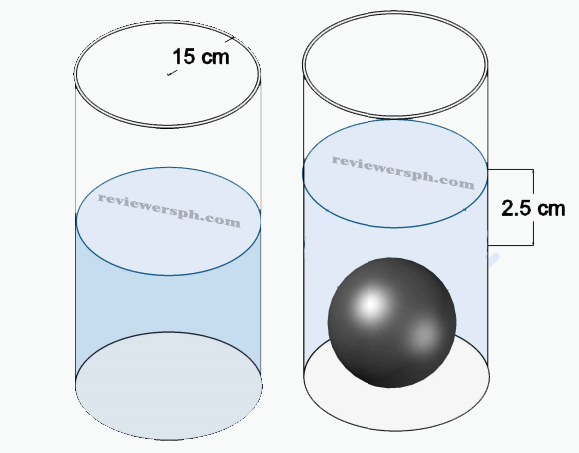### Math Notes

Subjects

#### High School Mathematics Solutions

##### Topics || Problems

A cylindrical bottle with radius 15cm is half-half filled with water. A spherical stone is dropped inside the bottle (completely submerging into the water) and the water level rises by 2.5 cm without spilling over. What is the radius of the stone?Volume of Cylinder, $$V_c = 2 \pi r^2 h$$

Volume of a sphere, $$V_s = \frac{4 \pi r^3}{3}$$

By principle of buoyancy, we are sure that the volume of the submerged object is equal to the volume of displaced water.

Thus, Volume of displaced water = Volume of Stone

$$V_w = 2\pi r^2h$$

$$V_w = 2\pi (15)^2(2.5) = 1125 \pi cc$$

$$V_{stone} = \frac{4\pi r^3 }{3}$$

$$1125 =\frac{4\pi r^3 }{3}$$

$$r = 9.45 cm$$